Skip to main content

# Dynamic time slot allocation and stream control for MIMO STDMA in ad hoc networks

## Abstract

Multiple-input multiple-output (MIMO) technologies have been shown to potentially improve the performance of network. Many traditional medium access control (MAC) protocols, such as spatial time division multiple access (STDMA), may not support MIMO technologies directly and not make full use of the feature of MIMO, which may be a limitation in the practical application in ad hoc networks. Therefore, in this paper, we propose a dynamic time slot allocation and stream control for MIMO STDMA (DTSMS) protocol to improve STDMA in performance. Utilizing stream control technology of MIMO and reservation scheme, DTSMS makes improvement on network throughput and avoids the mutual interference of neighbor links. Thus, DTSMS can support both unicast and multicast simultaneously. Moreover, we implement the dynamic time slot allocation scheme in DTSMS to guarantee the transmission efficiency of packets with high cross-layer transmission parameters, such as the packet priority, neighbor node density, or link quality. Utilizing the collected cross-layer information, the proposed scheme allocates time slots for all nodes dynamically according to the changes of network topology and nodes’ transmission parameters. Finally, the effectiveness of the protocol is demonstrated by numerical analysis and simulations. The results show that DTSMS outperforms STDMA in terms of network throughput and delay. Furthermore, compared to our previous TTS-MIMO, DTSMS can decrease the delay of packets with high transmission parameters and improve the quality of service (QoS).

## Introduction

Ad hoc networks are an infrastructureless networks where nodes are often inexpensive off-the-shelf equipment with wireless transceivers, which could be organized anywhere at any time [1, 2]. Ad hoc technology is widely used and has become the key technology in many fields, such as social and biological networks. Many networks succeeded in the recent years due to the development of ad hoc wireless technology , which obtains more and more attention. Nowadays, most of the medium access control (MAC) protocols in ad hoc networks are based on carrier-sense multiple access with collision avoidance (CSMA/CA) technology, but when the node’s quantity in a network is great and the network traffic is heavy, CSMA/CA protocol, as a competitive multiple access protocol, cannot satisfy the quality of service (QoS) requirements well. We are considering utilizing time division multiple access (TDMA) mechanism to reduce collisions and improve network throughput.

In general, MIMO technologies, which refer to using multiple related or unrelated antennas to send or receive data, make full use of the space dimension of communication system, broaden the application of traditional quantitative Shannon, and provide a new method to utilize the antenna arrays. MIMO technologies at the physical layer have been widely studied and applied in ad hoc networks; however, the advantages of MIMO, such as flexibility and network throughput improvement, could be utilized effectively only by designing appropriate upper-layer protocols. Moreover, with the advent of 5G technology [4, 5], the effective upper-layer protocols are significant to increase the capacity, reduce latency, and improve the energy efficiency of current mobile networks . Therefore, to design better MAC protocols supporting MIMO for ad hoc networks is essential for the rapid development of MIMO technologies. There are many classes of MIMO MAC protocols, such as stream control-based , encoding-based , and beamforming-based . We mainly focus on MIMO MAC protocols based on TDMA in this paper.

Traditional TDMA MAC protocols cannot fully multiplex channels, so spatial time division multiple access (STDMA) protocol in  is proposed to make full use of the channel of the network. STDMA is the extension of TDMA, which enables long-distance mobile nodes to share the same time slot to improve the throughput of network. In STDMA, space is divided into several virtual space slots and there are many nodes in each virtual space slot. If the distances between nodes are large enough, these nodes can transmit data in the same time without collision. In this way, STDMA can implement spatial reuse and increase network capacity. There have been many researches on the STDMA protocols in ad hoc networks [11, 12]. However, these protocols utilize the single-input single-output (SISO) technology. In addition, most of the MIMO MAC protocols are used for either unicast or multicast instead of supporting both simultaneously, which could be a limitation in practical applications.

Over the past years, a variety of aspects of MIMO MAC protocols in ad hoc networks have been studied. The authors in  propose centralized and distributed stream-controlled medium access (SCMA) protocols in order to maximize network resource utilization and ensure the fairness of network. However, SCMA protocol costs much to obtain the whole network topology, and furthermore, nodes’ mobility reduces its efficiency. The authors in  try to use spatial multiplexing and beam-forming technologies to improve network capacity. In , the authors propose a spatial diversity MAC (SD-MAC), which uses space-time block codes to obtain diversity gain and reduce the effect of channel fading. The authors in  propose a mitigating interference using multiple antennas (MIMA) protocol which uses a cross-layer design between MAC layer and physical layer  to reduce interference and improve network throughput. However, every transmission just uses half antennas in MIMA, which is a limitation that MIMA cannot adjust antenna numbers according to different scenarios.

The study of MIMO MAC taking into account TDMA is pioneered by [17, 18]. The authors in  use MIMO-OFDM technology and space-time block codes to achieve parallel transmission, but busy tone channel cost additional overhead. The protocol in  allocates each node time sub-slots to reserve and raises time slot utilization using MIMO technology. Moreover, cross-layer MAC design [15, 19, 20] is also used to improve the performance of networks. To break the strict limitation of the communication between layers, cross-layer MAC design fully considers the relationship of each layer and allows exchanging information between layers on the basis of the original protocol stacks . In terms of dynamic resource allocation, there are many existing methodologies to allocate the network resources (e.g., bandwidth , power per subcarrier , time slot and subchannel ) for nodes in the networks, which enhance the QoS and guarantee the packet transmission efficiently. Therefore, on the one hand, we can use the cross-layer information to adjust the transmission strategy adaptively to satisfy requirements of high layer, and on the other hand, we can also utilize them to implement time slot allocation for all the nodes in ad hoc networks. However, most of the existing time slot allocation schemes in ad hoc networks do not take the cross-layer information and the changes of network topologies [25, 26], which have the poor adaptability to network topology changes and cannot guarantee the QoS of the packets with high cross-layer transmission parameters.

Therefore, the main problem we address and the corresponding contribution of this paper is implementing a multiple access protocol in ad hoc network based on STDMA supporting MIMO, which can be divided into the following aspects specifically:

• Because most of MIMO MAC protocols are utilized for either unicast or multicast, in this paper, we propose a new MIMO STDMA (DTSMS) protocol using a stream control scheme. We implement three kinds of CTS packets to control the number of data streams to send data aimed at different cases; in this way, DTSMS protocol can support unicast and multicast simultaneously and improve the performance of the STDMA protocol.

• We implement dynamic time slot allocation scheme in DTSMS protocol to adjust the transmission time slots for all nodes according to the changes of network topologies and cross-layer information. Thus, DTSMS can decrease transmission delay of packets with high cross-layer transmission parameters (e.g., the video information in the city center) and improve the QoS further.

The rest of this paper is organized as follows. In Section 2, we describe the MIMO model and network model. The proposed protocol is described in detail, and some communication scenarios are presented in Section 3. In Sections 4 and 5, we evaluate the network performance of the DTSMS protocol through analysis and simulations, respectively. Finally, the conclusion is drawn and future works are also discussed in Section 6.

## System model

### MIMO model

Senders and receivers are equipped with multiple antennas in MIMO system, which can obtain diversity gain, multiplexing gain, and interference suppression ability to improve transmission performance. MIMO antennas are usually modeled by spatial degree of freedom (DOF). For a node with M antennas, it is generally considered to own M DOFs. For a MIMO link, its spatial degree of freedom is defined as the smaller DOF of the node on both sides of the link. For instance, the degree of freedom for the link, in which the DOF of sender is M s and the DOF of receiver is M r , is min(M s ,M r ). In the case of no interference streams, the link owning min(M s ,M r ) DOFs can transmit at most min(M s ,M r ) data streams. If interference streams existed, a part of DOF owned to receiver is used to suppress interference streams , so the number of data streams that the link can transmit will be less than min(M s ,M r ). In general, suppressing interference streams is done by the receiver. For example, a node owns M r DOFs can receive K s data streams and suppress K j interference streams successfully if they satisfy K s + K j M r . Without loss of generality, node i can receive all data streams, if the sum of data streams and interference streams sending to node i is not greater than the DOF of node i, that is $$\sum \limits _{s} {K_{s}} ~+~ \sum \limits _{j} {K_{j}} ~\le ~ {M_{i}}$$.

MIMO can provide stream control gain for MAC layer protocols. Assuming that each node owns M DOFs, each link also owns M DOFs. If there are m interfering links, maximum data streams transmitted on each link are K = M/m (M/m means the integer part of M/m), which is available to be employed to suppress interference streams. The gain achieved through such stream control is termed as stream control gain. In STDMA mode, for example, two links are arranged in different time slots, and each link uses M data streams (e.g., each stream is 25 kbps) for transmission. However, in stream control mode, these two links choose the best two streams (e.g., each stream is 30 kbps) to transmit in the same time slot, which provides an improvement about 20% in network capacity (120 kbps), compared to that in the TDMA mode (100 kbps). It has been proved that performing stream control mode could obtain up to 65% improvement in performance over the STDMA mode when measured at indoor channels in the presence of low correlation.

### Network model

We assume that an ad hoc network consists of N nodes, where each node can be denoted as iN. Node i has interference with node j if the signal-to-noise ratio (SNR) on the link from node i to node j is greater than or equal to a certain threshold , which is expressed by formula (1),

$$SNR(i,j) = \frac{P_{i}}{{L_{b}}(i,j){N_{r}}} \ge {\gamma_{0}},$$
(1)

in which P i indicates the transmission power of node i, and the path loss of this power to node j is denoted as L b (i,j). N r indicates the effect of the thermal noise. If the link from node i to node j satisfied (1), we say that node i and j are in the same contention area. As nodes in the network are distributed sparsely, several separated contention areas may exist according to the space.

A network topology of 19 nodes is shown in Fig. 1, and areas A, B, and C are three contention areas. Contention area is defined as a special area where nodes cannot use the same time slot to send data, such as nodes 6, 7, and 8 in area A. Nodes in different contention areas can be reused in the same time slot, such as nodes 7, 10, and 15, which belong to areas A, B, and C, separately. In each contention area, there is a center node which controls the time slot allocation in the contention area where it is allocated. In order to reduce the interference, the distance between center nodes in different contention areas must reach to a certain value. In Fig. 1, nodes 1, 2, and 3 are center nodes and satisfy the condition above.Fig. 1

## DTSMS protocol

The dynamic time slot allocation and stream control for MIMO STDMA (DTSMS) protocol is based on STDMA, which is a wireless MAC protocol for ad hoc networks, and it enables long-distance mobile nodes to share the same time slot for the purpose of improving network throughput.

The DTSMS protocol aims to utilize cross-layer information, such as traffic arrival rate, neighbor node density, and packet priority, to provide nodes with dynamic time slot allocation and support both unicast and multicast at the same time. The protocol could satisfy the QoS requirement in terms of delay, and network throughput is also improved by exploiting MIMO technology.

In order to satisfy the QoS requirement, each node needs to send HELLO packets in each cycle to obtain neighbor node density. Here, a cycle is defined as the period that consists of allocated time slots of all nodes. Then, each node sends cross-layer information, including traffic arrival rate, neighbor node density, and packet priority to the center node, as a basis to decide time slot allocation. Therefore, time slot allocation is considered about the service’s characteristics and is also adjusted periodically and dynamically according to the changes of network topologies and the cross-layer information. In this way, the higher the packet priority, the higher the neighbor node density, and the better the link quality a node has, the earlier the node sends data packets.

Besides that, nodes could use clear channel assessment (CCA) technology to occupy idle time slots and send packets in order to avoid the waste of time slots. Network throughput is also improved with collision resolution by exploiting MIMO technology. The details of the protocol are described in the following subsections.

### Stream control scheme

We consider node S as a sending node and node R as a receiving node; each node owns M DOFs. For the purpose of taking full advantages of MIMO, stream control scheme is proposed. By using several types of CTS packets, we can choose the number of data streams to transmit data. CTS packets have three types which are used in three different cases, respectively. The three kinds of CTS packets are described as follows:

1. 1.

The CTS I packet is used when node R only receives 1 RTS packet for itself without hearing any other RTS packets from other nodes at the same time. In this case, node R replies to a CTS I packet to node S to inform it to use all DOFs for this data transmission.

2. 2.

The CTS II packet is used when node R receives N RTS packets for itself without hearing any other RTS packets from other nodes at the same time. In this case, node R replies to CTS II packets to inform nodes who send RTS packets to node R that they could use M/N DOFs for this data transmission.

3. 3.

The CTS III packet is used when node R not only receives N RTS packets for itself but also hears other RTS packets from other nodes at the same time. In this case, node R replies to CTS III packets to inform nodes who send RTS packets to node R that they could just use one DOF for this data transmission. RTS packets, CTS packets, or ACK packets are sent by only one DOF, which is good for avoiding collision.

### Dynamic time slot allocation scheme

In order to shorten the average packet queuing delay further, we are going to consider neighbor node density and packet priority. After choosing proper time slot length, we make use of neighbor node density and packet priority to adjust time slot allocation to satisfy QoS requirement better.

In the DTSMS protocol, we choose neighbor node density in the network layer, packet priority in the MAC layer, and link quality in physical layer as three factors which could decide the time slot allocation, since these three factors are related to the performance of unicast and multicast. The packets of higher priority need to be transmitted in a lower delay, and the packets of lower priority may not care about delay. Each node sends neighbor node density and packet priority to the center node, and then, the center node calculates the Q i according to formula (2). According to the Q i , the time slot allocated to a node is determined and changes with the topology of network.

$${Q_{i}} = {p_{i}}{\omega_{p_{i}} + {d_{i}}{\omega_{d_{i}}}+ {l_{i}}{\omega_{l_{i}}}},$$
(2)

where p i , d i , and l i are the priority, neighbor node density, and link quality of the ith node, respectively. $$\omega _{{p_{i}}}$$, $$\omega _{d_{i}}$$, and $$\omega _{l_{i}}$$ are the normalization coefficients of the priority, neighbor node density, and link quality.

#### The cross-layer information weight determining mechanism

In order to get the expression of the transmission factor, we need to calculate the normalization weight of the packet priority, neighbor node density, and link quality, respectively. In this way, we build the straight reciprocal matrix of weight by comparing the influence to data transmission of any two of the influence factors. Then, we use power method  to calculate the maximum eigenvalue and its eigenvector of the matrix. After we normalize the eigenvector, we can get $$\omega _{p_{i}}$$, $$\omega _{d_{i}}$$, and $$\omega _{l_{i}}$$. The process can be presented as follows:

According to the impact of the cross-layer information to packet transmission, we can build a 33 straight reciprocal matrix, and every element in the matrix is the weight ratios of any two of the cross-layer information. Actually, the accurate weight ratios of the cross-layer information are hard to be estimated which can be chosen according to the actual requirements and users’ preference. Therefore, we take an example to indicate the build process of the straight reciprocal matrix which can be presented as formula (3),

$$A= \left[ \begin{array}{cccc} \frac{\omega_{1}}{\omega_{1}} & \frac{\omega_{1}}{\omega_{2}} & \frac{\omega_{1}}{\omega_{3}}\\ \frac{\omega_{2}}{\omega_{1}} & \frac{\omega_{2}}{\omega_{2}} & \frac{\omega_{2}}{\omega_{3}}\\ \frac{\omega_{3}}{\omega_{1}} & \frac{\omega_{3}}{\omega_{2}} & \frac{\omega_{3}}{\omega_{3}} \end{array} \right] = \left[ \begin{array}{cccc} 1 & \frac{3}{2} & 2\\ \frac{2}{3} & 1 & \frac{6}{5}\\ \frac{1}{2} & \frac{5}{6} & 1 \end{array} \right],$$
(3)

where ω 1, ω 2, and ω 3 are normalization coefficients of the priority, neighbor node density, and link quality, respectively.

Because matrix A is a positive reciprocal matrix whose maximum eigenvalue λ max is the simple multiplicity of eigenvalues. For the other eigenvalues λ, they all follow:

$$\lambda_{max} > |\lambda|.$$
(4)

Given the eigenvalues of the N-order matrix are λ 1, λ 2,…, λ n , where

$$|\lambda_{1}| > |\lambda_{2}| > \ldots > |\lambda_{n}|.$$
(5)

And the relevant linearly independent eigenvectors are u 1, u 2,…,u n . Thus the n eigenvectors can be a set basis of R n. In this way,

$$\forall x^{0} \in R^{n}, \quad \quad x^{0} = \sum\limits_{i=1}^{n} \alpha_{i} u_{i},$$
(6)

where α i presents the ith coordinate under this basis.

Then, we can use the iteration formula (7) to obtain the eigenvector.

$$x(k+1) = A x(k) \quad k = 0,1,...$$
(7)

Thus, we can get:

$$x(1) = Ax(0) = A\sum\limits_{i=1}^{n} \alpha_{i} u_{i} = \sum\limits_{i=1}^{n} \alpha_{i} \lambda_{i} u_{i},$$
(8)
$$x(2) = Ax(1) = A^{2}x(0) = A \sum\limits_{i=1}^{n} \alpha_{i} \lambda_{i} u_{i} = \sum\limits_{i=1}^{n} \alpha_{i} \lambda_{i}^{2} u_{i},$$
(9)
\begin{aligned} x(k) & = A^{k}x(0) = \sum\limits_{i=1}^{n} \alpha_{i} A^{k} u_{i} = \sum\limits_{i=1}^{n} \alpha_{i} \lambda_{i}^{k} u_{i} \\ &= \lambda_{1}^{k} \left[\alpha_{1}u_{1} + \sum\limits_{i=2}^{n} \alpha_{i} \left(\frac{\lambda_{i}}{\lambda_{1}}\right)^{k} u_{i}\right] \approx \lambda_{1}^{k}\alpha_{1}u_{1}. \end{aligned}
(10)

### Proof

According to formula (10), when k = 2,3,…, we can get

$$x(k) = A^{k}x(0) = \lambda_{1}^{k} (\alpha_{1}u_{1}+ \varepsilon_{k}),$$
(11)

where $$\varepsilon _{k}~=~\alpha _{2}\left (\frac {\lambda _{2}}{\lambda _{1}}\right)^{k} u_{2} + \ldots + \alpha _{n}\left (\frac {\lambda _{n}}{\lambda _{1}}\right)^{k} u_{n}$$. Moreover, according to the assumption |λ 1|>|λ 2|>…>|λ n |, we can get

$$\left|\frac{\lambda_{i}}{\lambda_{1}}\right|<1 \quad i = 2,3,\ldots,n.$$
(12)

Therefore, we can get

$${\lim}_{k \to \infty}\left(\frac{\lambda_{i}}{\lambda_{1}}\right)^{k} = 0 \quad i = 2,3,\ldots,n.$$
(13)

In this way, we can get the equation

$${\lim}_{k \to \infty}\varepsilon_{k} = 0.$$
(14)

And the speed of convergence depends on the ratio $$r = \left |\frac {\lambda _{2}}{\lambda _{1}}\right |$$, and the smaller the r is, the faster the convergence speed will be. Therefore, when k is large enough, we can get

$$x(k) = \lambda_{1}^{k} \alpha_{1} u_{1}.$$
(15)

On the one hand, if |λ 1|>1, when k is large enough, $$\left |\lambda _{1}^{k}\right |$$ will be very large which cause some difficulties to the calculation. On the other hand, if |λ 1|<1, when k is large enough, $$\left |\lambda _{1}^{k}\right |$$ will be very close to 0 which is also a problem to the calculation. Therefore, we can follow the formula (16) by transforming the vector after each iteration into the vector whose maximum component is 1.

$$\left\{ \begin{array}{lcl} \beta = \max\{ x(k)_{i} | i=1,2,\ldots,n \} \\ y(k) = \frac{1}{\beta}x(k) \\ x(k+1) = Ay(k) \end{array} \right.$$
(16)

When |β k+1β k | is smaller than ε, β k is the approximate value of the absolute value of the maximum eigenvalue after the kth iteration and x(k + 1) is the relevant eigenvector. We assign ε as 0.001. Thus, as Table 1 shows, when |β k + 1β k | < 0.001, we consider the maximum eigenvalue has met our requirements.

Finally, we normalize the eigenvector x(k + 1), and the normalized eigenvector is

$$[0.4626, 0.2978, 0.2396].$$
(17)

Therefore, the expression of transmission factor Q i is

$$Q_{i} = 0.4626p_{i} + 0.2978d_{i} + 0.2396l_{i}.$$
(18)

### Definition 1

Equivalent nodes: Two nodes in the same contention area have the same connectivity with the other nodes. And if node i and node j are equivalent, which can be presented as

$$\left\{ \begin{array}{lcl} \delta_{ii} = \delta_{jj} = 1 \\ \delta_{ij} = \delta_{ji} \\ \delta_{in} = \delta_{jn} \quad (n \neq i,j) \end{array}. \right.$$
(19)

Take the network topology in Fig. 1 as an example; according to the definition, we can get three equivalent node groups [6, 7, 8], [13, 14], and [16, 17, 18]. Moreover, in order to make use of STDMA, all the nodes should be allocated into different time slots and the nodes in the same contention area should not be allocated in the same time slot.

Therefore, all the 19 nodes in the network can be divided into six time slots, and all the feasible schemes can form a set U. At first, we should choose one allocation scheme from U to send data randomly. Then, if the network topology does not change, hub will record the transmission factor Q of transmission nodes and adjust the transmission sequence among the equivalent node groups according to the transmission factor Q. The larger the transmission factor Q of the transmission nodes are, the earlier the data in the nodes will be transmitted. However, if the network topology changes, the hub should calculate the average accumulated transmission factor E[ Q] of all the time slots. The average accumulated transmission factor E[ Q j ] of the jth time slot can be presented as

$$E[\!Q_{j}]=\frac{1}{m_{j}}\sum\limits_{i~=~1}^{m_{j}}Q_{i},$$
(20)

where m j is the number of transmission nodes is the jth time slot. Finally, the hub will calculate the scheme parameter K of all the allocation schemes in U according to formula (21) and choose the allocation scheme with the minimum scheme parameter K as the new time slot allocation scheme. The process is presented in Algorithms 1.

$$K=\sum\limits_{j=1}^{6}jE[Q_{j}].$$
(21)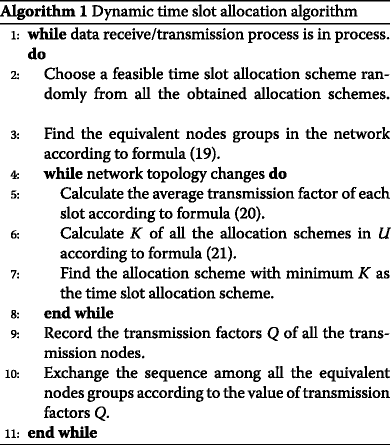### Communication process description

Firstly, each node sends traffic arrival rate to the center node, which calculates t slot1 and t slot2 with the QoS requirement. t slot1 is the minimum time slot length when slot utilization reaches maximum α max, and t slot2 is the maximum time slot length when average packet queuing delay satisfies the QoS requirement approximately. If t slot1t slot2 as the later discussion shows, we will choose t slot1 as the time slot length; thus, slot utilization is maximum and average packet queuing delay is lower. And if t slot1 > t slot2, we will choose t slot2 as the time slot length, because we choose to satisfy the QoS requirement about delay at the cost of slot utilization.

Secondly, each node in the network sends one-hop HELLO packets periodically to get its neighbor node density. Then, each node sends the neighbor node density and packet priority to the center node, which generates time slot factor Q i through formula (2). According to the Q i , center node allocates time slots to every node. The more neighbor nodes and the higher packet priority a node has, the larger the Q i is, so the earlier time slot will be allocated to the node. We allocate time slot in this way due to that neighbor node density is a factor to decide the performance of multicast, and packet priority is a factor to decide the performance of both multicast and unicast. In other words, the more neighbor nodes and the higher packet priority a node has, the earlier the node could send packets.

Then, in the time slot allocated to node S, if the traffic is unicast, the node S sends RTS packet directly, and if the traffic is multicast, the node S sends packet of multicast directly using full antennas. In the idle time slot unallocated to node S, the node S reserves channel to realize unicast or sends data packet of multicast using one antenna. According to types of receiving CTS packet, node S selects appropriate number of streams to send data packets. When a data packet of unicast is received successfully, node R replies ACK packet to node S for confirmation. Otherwise, a failed transmission occurs and node S needs to retransmit the data packet. ACK packet is unnecessary in multicast transaction. The operation process of node S and node R is shown in Algorithms 2 and 3, respectively.

We take the transmission case in the slot 1 of area A as a specific instance to analyze operation procedure in DTSMS, and the operation procedure in other contention areas are similar as this instance.

If the node is in a contention area, such as node 7, the node can receive 1 RTS packets and send data using all the four antennas. However, if the node is in different contention areas, such as node 5, it can receive many RTS packets or multicast packets and utilize the corresponding CTS packets to control the number of data streams.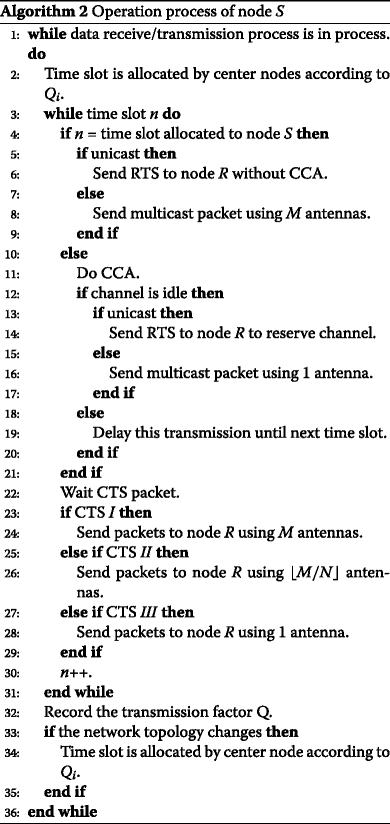As shown in Fig. 2, when node 6 sends data packets in its own slot using unicast, node 6 can send RTS packet directly in the slot. Thus, node 7 can only receive one RTS and cannot hear any other RTS packets from other nodes in the same time slot. Node 7 replies CTS I packet to node 6 and indicates that node 6 can transmit data using four antennas. When node 7 receives data packets from node 6 successfully, node 7 replies ACK packet to node 6. Meanwhile, other nodes will keep quiet in this time slot until the next time slot.Fig. 2

Figure 3 shows the scenario where node 6 has no data packet to send in its allocated time slot. However, node 7 and node 9 have data packets of unicast to send to node 8 in this slot. After they do CCA and find the channel is idle, they send RTS packets to make reservation. Thus, node 8 will receive 2 RTS packets and reply a CTS II packet to nodes 7 and 9 notifying them to send two parallel data streams, respectively. In this way, the idle time slots can be utilized, but also, the process can make full use of the interference suppression in MIMO technologies to avoid collision efficiently.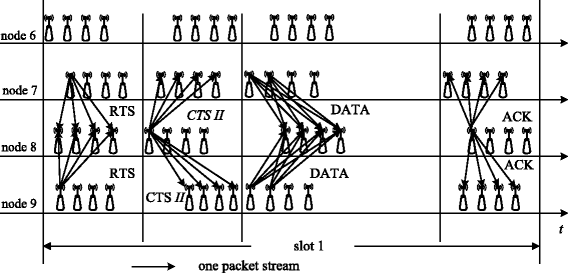Fig. 3

Figure 4 shows the transmission procedure that the receiving node not only receives RTS packet for itself but also hears other packets for other nodes at the same time. If node 6 has no packet to send in its allocated slot and node 8 and node 4 have data packets to send to node 9 and node 5, respectively, then they will send RTS packets to make reservation. However, in this case, node 9 will not only receive a RTS packet from node 8 but will also hear a RTS packet from node 4. Therefore, in order to avoid collision, node 9 and node 5 send CTS III packets to node 8 and node 4 to notify them to send one data stream, respectively.Fig. 4

### Scenarios

We make some assumptions regarding the previous subsections of DTSMS:

1. 1.

The network topology is shown in Fig. 1, and each node has 4 DOFs in the network.

2. 2.

All the nodes keep moving in the network and the positions of node 1, node 2, and node 3 are almost unchanged relative to other nodes.

3. 3.

Nodes within one-hop range are mutual-communicated, and nodes within two-hop range are mutual-sensed.

4. 4.

The node 1, node 2, and node 3 are center nodes in each contention area. The cross-layer information can be transmitted to the center nodes to calculate the transmission factors of transmission nodes according to formula (2).

5. 5.

According to the transmission factors of every node, the time slots are allocated dynamically.

## Performance analysis

In this section, we analyze the throughput and delay of our proposed protocol under unsaturation condition. We take the nodes in area A as a specific instance to analyze the performance of the protocol because the operation procedure in other contention areas is similar as in this instance. Moreover, we consider every node is only in one contention area. That is to say, the nodes which are located in the different contention areas are ignored. Table 2 lists the important notation that is used in this paper.

### Throughput

Suppose there are N nodes in the network and every node has at least M different packets to send simultaneously by using all M DOFs at the start of its own slot. However, if some slots which have been allocated to nodes are idle, other nodes may occupy the idle slot to send packets. The number of antennas used to send packets depends on the kind of the RTS packet it receives.

Let S be the network throughput, P be the total received packets size, and τ be the total transmission time, then

$$S = \frac{P}{N\tau}.$$
(22)

According to the stream control model, P consists of two parts: the packet size P in sent by the owner of this time slot and P out by other nodes if the owner of this time slot has no packets to send.

For one thing, if the node has data packets to send in the allocated time slot, according to the operation process in the system model, it can use all M DOFs to send packets. Let P n be the packet size of the nth packet, λ be the traffic arrival rate, and N t be the number of received packets; the total received packet size P in using 1 antenna can be shown as

$$P_{in} = \sum\limits_{n=1}^{N_{t}} P_{n}.$$
(23)

The mathematical expectation of total received packet size can be shown as

\begin{aligned} E[\! P_{N_{t}}] & =\! E[E[P_{N_{t}}|N_{t}]] \\ & = \sum\limits_{k=1}^{\infty}E[\! P_{N_{t}}|N_{t} = k]p(N_{t} = k)\\ & = E(P)\sum\limits_{k=1}^{\infty}k * p(N_{t} = k)\\ & = E(P)*\lambda\tau, \end{aligned}
(24)

where E(P) presents the average packet size.

Moreover, the DOFs of the process is M, and the mathematical expectation of P in is

$$P_{in} = M*E(P)*\lambda\tau.$$
(25)

For another, if the node has no packets to send in its allocated time slot, the number of antennas used to send packets is different in this process. Let N be the number of nodes in a cluster, V be the number of requested antennas and p be p(N t = 0). If there is only one node sending packets, in this case, according to the operation process, this node will receive the CTS I packet and use M antennas to send packets. The probability of this case can be presented as

\begin{aligned} p(V = M) &= \binom{1}{N-1}p^{N-2}(1 - p) \\&= (N - 1)e^{-\lambda\tau(N-2)}(1 - e^{-\lambda\tau}). \end{aligned}
(26)

If the receiving node has receive k RTS packets for itself and not heard any other RTS packets from other nodes at the same time, in this case, it will reply a CTS II packet to the k sending nodes and the sending nodes will send packets using M/k antennas. The probability of this case can be presented as

\begin{aligned} p(V & = \left\lfloor {M/k} \right\rfloor)\\ & = \binom{k}{N-1}p^{N-1-k}(1 - p)^{k}\left(\frac{1}{N}\right)^{k-1} \\ & = \binom{k}{N-1}e^{-\lambda\tau(N-k-1)}(1 - e^{-\lambda\tau})^{k}\left(\frac{1}{N}\right)^{k-1}. \end{aligned}
(27)

If the receiving node has not only received RTS packet for itself but also heard other packets for other nodes at the same time, in this case, it will reply a CTS III packet to the sending node to notify it to send one data stream. The probability of this case can be presented as

\begin{aligned} p(V & = 1)\\ & = \sum\limits_{l~=~2}^{M}\left[\binom{l}{N~-~1}e^{-\lambda\tau(N~-~l~-~1)}(1 - e^{-\lambda\tau})^{l}\left[1-\left(\frac{1}{N}\right)^{l}\right]\right]. \end{aligned}
(28)

Therefore, the mathematical expectation of the number of antennas V can be presented as

\begin{aligned} E(V) & = M (N - 1)e^{-\lambda\tau(N-2)}(1 - e^{-\lambda\tau}) \\ & + \left\lfloor {M/k} \right\rfloor \binom{k}{N-1}e^{-\lambda\tau(N-k-1)}(1 - e^{-\lambda\tau})^{k}\left(\frac{1}{N}\right)^{k-1} \\ & + \sum\limits_{l=2}^{M}\left[\binom{l}{N-1}e^{-\lambda\tau(N-l-1)}(1 - e^{-\lambda\tau})^{l}\left[1-\left(\frac{1}{N}\right)^{l}\right]\right]. \end{aligned}
(29)

Moreover, the mathematical expectation of P in is

$$P_{out} = E(V)*E(P)*\lambda\tau.$$
(30)

Above all, the throughput can be presented as

\begin{aligned} S & = \frac{(P_{in} + P_{out})(1~-~p)p}{N\tau} \\ & = \frac{[M\lambda E(P)(1~-~p)~+~E(V)\lambda E(P)(N~-~1)](1~-~p)p}{N}. \end{aligned}
(31)

### Delay

We use the queue theory to analyze the delay of our DTSMS protocol. In this protocol, we use STDMA protocol to allocate the time slots for every node in ad hoc networks; therefore, the nodes can send their packets in their allocated time slots and sleep in other time slots. In this way, we can use M/G/1 queues with generalized vacations to analyze the delay of this process.

As shown in Fig. 5, suppose when the ith packet arrives, there are N i packets in the queue, and the lth packet is receiving services and its remaining service time is R i . Let the service time of the kth packet be X k and the waiting transmission time of other nodes be Y i ; therefore, the waiting time of the ith packet is

$$W_{i} = R_{i} + \sum\limits_{k~=~i~-~N_{i}}^{i~-~1}X_{k}~+~Y_{i}.$$
(32)Fig. 5

Let the service rate be μ and the proportion of packet transmission $$\rho = \frac {\lambda }{\mu }$$; therefore, the expression of waiting time W is

$$W = \frac{R~+~Y}{1~-~\rho}.$$
(33)

As Fig. 6 shows, the shaded parts are the remaining service time r(t) of packet transmission and the other parts are the remaining service time of vacations.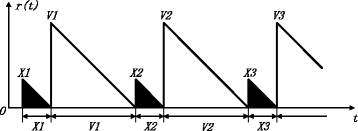Fig. 6

Suppose the M(t) and L(t) are the number of served users and vacations in the time periods [0, t], respectively, and the average remaining service time can be presented as

\begin{aligned} \frac{1}{t}\int_{0}^{t} {r(\tau)d\tau} & = \frac{1}{t}\sum\limits_{i=1}^{M(t)}\frac{1}{2}X_{i}^{2}+\frac{1}{t}\sum\limits_{i=1}^{L(t)}\frac{1}{2}V_{i}^{2} \\ & = \frac{1}{2} \frac{M(t)}{t} \frac{\sum\limits_{i=1}^{M(t)}\frac{1}{2}X_{i}^{2}}{M(t)} + \frac{1}{2} \frac{L(t)}{t} \frac{\sum\limits_{i=1}^{L(t)}\frac{1}{2}V_{i}^{2}}{L(t)}, \end{aligned}
(34)

where $$\frac {M(t)}{t}$$ is the average packet arrival rate, $$\frac {L(t)}{t}$$ is the average vacation arrival rate, $$\frac {\sum \limits _{i=1}^{M(t)}\frac {1}{2}X_{i}^{2}}{M(t)}$$ is the second moment of X i , and $$\frac {\sum \limits _{i=1}^{L(t)}\frac {1}{2}V_{i}^{2}}{L(t)}$$ is the second moment of V i .

The periods of packet transmission and vacations are full of the whole time, so the proportion of packet transmission is ρ and the vacation arrival rate is $$\frac {1~-~\rho }{\overline {V}}$$ where λ is the packets arrival rate and μ is the service rate. Let t, and the average remaining service time can be presented as

$$R=\frac{1}{2}\lambda\overline{X^{2}}+\frac{1}{2}\frac{1-\rho}{\overline{V}}\overline{V^{2}}.$$
(35)

However, if some nodes have no packets to be transmitted, the other nodes could occupy idle time slots and send packets. The remaining service time of this process is shown in Fig. 7. Suppose some nodes have no packets to be transmitted in vacations $$V_{1}^{\prime }$$ and $$V_{2}^{\prime }$$ and the node transmitting packets in the first time slot occupies the idle time slots and sends packets. However, as shown in Fig. 7, although the node occupies the same number of time slots in vacations $$V_{1}^{\prime }$$ and $$V_{2}^{\prime }$$, the remaining service time is different and the longer the continuous vacations are, the longer the remaining service time is. Moreover, the remaining service time is related to the number of time slots the node can occupy and the sequence of the occupied time slots.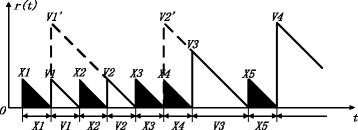Fig. 7

As we can see in Fig. 8, the first column in the figure means the number of occupied time slots and the second column illustrates the remaining service time with different occupied time slots and the probability of all the cases. It is important to note that every remaining service time diagram r(t) in the figure is just a typical example of each case and we do not list all the cases for simplicity. Moreover, the third column illustrates the value of r(t) corresponding to different number of occupied time slots where τ is the length of a time slot.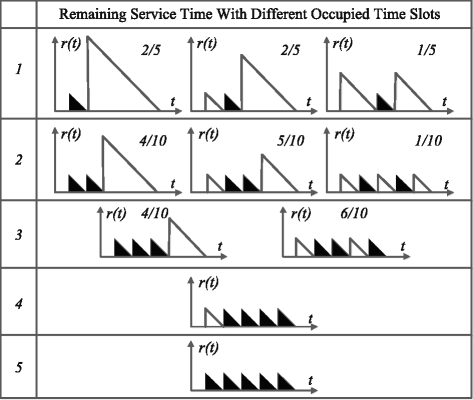Fig. 8

Therefore, according to the number of antennas used to transmit packets in Algorithm 2, the formula (35) can be presented as

\begin{aligned} R & = \frac{\frac{1}{2}\lambda\overline{X^{2}}}{M} + \frac{R^{\prime}}{E(V)} \\ &=\frac{\frac{1}{2}\lambda\overline{X^{2}}}{M}+\frac{\frac{1}{2}\frac{1-\rho}{\overline{V^{\prime}}}\overline{V^{{\prime}2}} + \frac{1}{2}\lambda\overline{X^{{\prime}2}}}{E(V)}, \end{aligned}
(36)

where R is the remaining service time during the period vacation time, $$\overline {V^{\prime }}$$ represents the average vacation time after the idle time slots occupation, and $$\overline {X^{\prime }}$$ represents the average transmission time during the primary vacation time.

Moreover, let V m and p(V m ) be the vacation time and the probability, respectively, of the mth line in Fig. 8, so the vacation time of the mth line $$\overline {V_{m}^{\prime }}$$ is

$$\overline{V_{m}^{\prime}}= m\tau.$$
(37)

Similarly, $$\overline {V_{m}^{\prime 2}}$$ can be presented as

$$\overline{V_{m}^{{\prime}2}}=\sum\limits_{n=1}^{k}p(mn)\overline{V_{mn}^{2}},$$
(38)

where $$\overline {V_{mn}^{2}}$$ and p(mn) are the second moment of vacation time and the probability of the nth diagram in the mth line, respectively, which have been labeled in Fig. 8.

Then, the average transmission time during the primary vacation time $$\overline {X_{m}^{{\prime }2}}$$ is

$$\overline{X_{m}^{{\prime}2}} = (5-m)\tau^{2}.$$
(39)

Above all, let r m be the remaining service time during the vacation time and we can get the expression of r m

$$r_{m} = \frac{1}{2}\frac{1-\rho}{\overline{V_{m}^{\prime}}}\overline{V_{m}^{{\prime}2}} + \frac{1}{2}\lambda\overline{X_{m}^{{\prime}2}}.$$
(40)

Therefore, the remaining service time during the period vacation time R in formula (36) can be presented as

\begin{aligned} R^{\prime} &= (1-e^{-\lambda\tau})^{5-m}\sum\limits_{m=0}^{5}(1-e^{-\lambda\tau})^{m}(e^{-\lambda\tau})^{5-m}r_{m}\\ &= (1-e^{-\lambda\tau})^{5}\sum\limits_{m=0}^{5}(e^{-\lambda\tau})^{5-m}r_{m}. \end{aligned}
(41)

Furthermore, when the ith packet arrives in the transmission time of the lth node, if the ith packet belongs to the (l + 1)th node, this packet needs to wait for one transmission time slot. However, if the ith packet belongs to the (l − 1)th node, this packet needs to wait for five transmission time slots.

Therefore, suppose the ith packet of the (l+j) mod 6 node arrives in the transmission time slot of the lth node, the waiting transmission time Y i = (j mod 6)τ when there are no idle time slots. However, if the transmission node occupies the idle time slots, the waiting transmission time will be shortened. The average waiting transmission time Y is

$$Y = \frac{1}{6}\sum\limits_{m=1}^{5}Y_{i} = \frac{1}{6}\sum\limits_{m=1}^{5}(1-e^{-\lambda\tau})^{m}\sum\limits_{j=0}^{m}(m-j)\tau.$$
(42)

Putting formula (36), formula (41), and formula (42) into formula (33), the average waiting time can be presented as

$$W = \frac{1}{1-\rho}\left[\frac{\frac{1}{2}\lambda\overline{X^{2}}}{M}+\frac{R^{\prime}}{E(V)}+Y\right].$$
(43)

## Simulations

In order to verify the validity and effectiveness of DTSMS, in this section, we finish some simulations according to the protocol we propose above in MATLAB. Firstly, based on the network topology in Fig. 1 and the analysis results in Section 5, we compare the network throughput and delay of DTSMS with that of STDMA and TTS-MIMO . Then, we compare the transmission factor Q and the cross-layer information of all the time slots under the same scenario to verify the effectiveness of the dynamic slot allocation algorithm. Moreover, we record the slot utilization ratio of DTSMS and STDMA under different number of transmission users and transmission chances. The MAC parameters are listed in Table 3.

### Simulation 1: throughput with different traffic arrival rates

Figure 9 shows the unsaturated throughput with different traffic arrival rates under different packet sizes. According to the network topology in Fig. 1, there are six transmitters in a contention area and each transmission stream is 200 bytes in this simulation scenario. The DOF M of each node is 4. As we can see in the line chart, the unsaturated throughput of DTSMS is higher than that of STDMA because of the stream control scheme and idle slot occupation. Moreover, the throughput of DTSMS is 14.3% higher than that of TTS-MIMO  because the dynamic time allocation scheme increases the transmission chances of the packets with high link quality which decrease the retransmission times and improve the throughput of DTSMS. We record the analysis results and compare them with the simulation results; however, there are gaps between the analysis results and the simulation results. Comparing the simulation scenario to the analysis scenario, we always take all the scenarios into consideration in analysis scenario. For examples, there are no packet transmitted in all the time slots and the neighbor node density is quite low and these scenarios will decrease the performance of DTSMS. However, these scenarios do not happen frequently in the simulation scenarios and in real lives; therefore, there are gaps between the analysis results and the simulation results and the performance of simulation results are always better than that of analysis results.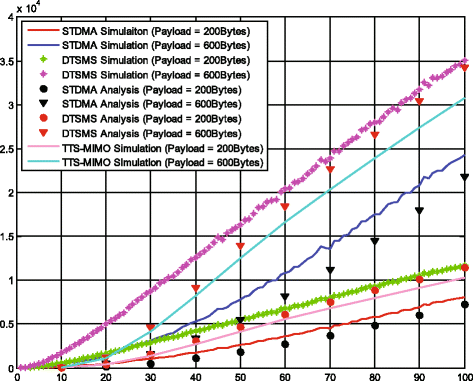Fig. 9

### Simulation 2: delay with different traffic arrival rates

Figure 10 shows the delay with different traffic arrival rates, and the simulation scenario is the same as that in simulation 1. As shown in Fig. 10, the delay of DTSMS is lower than that of STDMA because in the DTSMS protocol, transmitter will occupy the idle time slots if there are no packets to be transmitted in the slots, which could decrease the packet delay effectively. Moreover, the delay of DTSMS is 2.6% higher than that of TTS-MIMO  which means that the performance of DTSMS and TTS-MIMO is almost the same in terms of delay. This is because the dynamic time allocation scheme in DTSMS will cost some time to allocate time slots for all the nodes when the network topology or the sequence of transmission factors change. The analysis results are corresponding with the simulation results, which indicate the validity of our delay analysis.Fig. 10

### Simulation 3: priority and neighbor node density in DTSMS and TTS-MIMO

Figure 11 shows the average slot number of several priorities and neighbor node densities in DTSMS and TTS-MIMO, respectively. In general, in DTSMS and TTS-MIMO, the higher the priority of a packet is, the lower the normalized slot levels of the node is, and similarly, the larger the neighbor node density is, the lower the normalized slot levels of the node is. Moreover, when we compare the same priority and neighbor node density between DTSMS and TTS-MIMO, we can find that if the priority and normalized neighbor node density of a node are larger than three, the normalized slot level in DTSMS is lower than that of TTS-MIMO and if the nodes’ priority and neighbor node density is small, the normalized slot level in DTSMS is higher than that of TTS-MIMO. In other words, the normalized slot level of nodes with high transmission parameters in DTSMS are lower than that in TTS-MIMO, because the dynamic time slot allocation scheme will allocate low slots for the nodes with high transmission parameters, which will efficiently decrease the delay of the nodes with high transmission parameters and is useful for improving the quality of service.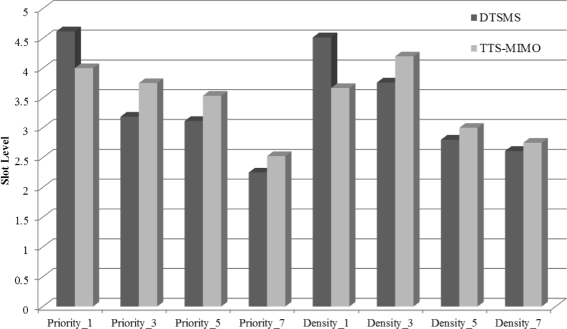Fig. 11

### Simulation 4: transmission factor and cross-layer information in different time slots

Figure 12 shows the transmission factor, priority, neighbor node density, and link quality in all the six time slots. The horizontal axis describes the slot sequence, and the vertical axis describes the normalized slot level; the higher the slot level is, the larger the transmission factor, priority, neighbor node density, and link quality are. As we can see in Fig. 12, the transmission factor decreases with the increase of the slot sequence which indicates the average delay of the packets with a large transmission factor is lower than that of the other packets with a small transmission factor. Moreover, the priority of each time slot also decreases with the increase of the slot sequence. However, the downward trends of neighbor node density and link quality are not obvious because the influence to packet transmission of priority is higher than that of the other cross-layer information. From this figure, we can draw that the DTSMS protocol brings QoS improvement in system by considering the cross-layer information of transmitters.Fig. 12

### Simulation 5: slot utilization ratio with different transmission chances

This simulation evaluates the relationship of average throughput per slot and number of transmitting users. There are 40 transmitters in a contention area, and each transmission stream is 200 bytes in this simulation scenario. The DOF M of each node is 4. We assume that every time slot is assigned to a proper time slot length to allow at most two transmission chances according to the QoS requirement. A cycle consists of all time slots allocated to nodes in a contention area, but only 20 nodes are transmitting and the other 20 nodes keep silence.

Figure 13 shows the average throughput per slot when each transmitter has 1, 2, and 4 transmission chances, respectively. In the STDMA protocol, each transmitter can use 4 DOFs to send data packets in their own slot without any collision. While in the DTSMS protocol, the transmitter not only sent data packets in their own slot but also allowed to send data packets in the unallocated slot when the slot is sensed to be idle. Therefore, the slot utilization ratio of DTSMS is higher than that of traditional STDMA.Fig. 13

### Simulation 6: Overhead in DTSMS and TTS-MIMO

Figure 14 shows the overhead in DTSMS and TTS-MIMO. The horizontal axis describes the transmission time, and the vertical axis describes the overhead of DTSMS and TTS-MIMO. As we can see in Fig. 14, the overhead of DTSMS is higher than that of TTS-MIMO because of the dynamic nature in dynamic time slot allocation scheme. However, from another perspective, the increase of overhead in DTSMS seems acceptable in terms of the quality of service improvement.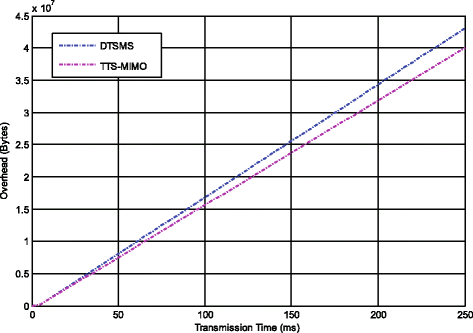Fig. 14

## Conclusions

Aiming to deal with the practical limitation in most of the MIMO MAC protocols, DTSMS protocol is proposed to improve the performance of the traditional STDMA in ad hoc networks. The DTSMS protocol highlights time slot allocation and stream control scheme, which could satisfy the QoS requirement in terms of delay and support both unicast and multicast at the same time. Considering the impact of cross-layer information, such as packet priority, neighbor node density, and link quality, DTSMS protocol decreases the delay of packets with higher transmission factor in ad hoc networks. The analysis and simulation results show that the performance of DTSMS and TTS-MIMO are better than that of STDMA. Moreover, although the delay and overhead of DTSMS are higher than those of TTS-MIMO because of the dynamic nature of DTSMS, the throughput of DTSMS is also higher than that of TTS-MIMO, which is acceptable in terms of the QoS improvement.

Some other significant cross-layer information could influence the performance of our DTSMS protocol, such as channel state information (CSI). Therefore, taking the other important cross-layer information into comprehensive consideration will be part of our future work, which highlights the advantages of our DTSMS protocol.

## References

1. 1

Y Song, J Xie, BRACER: a distributed broadcast protocol in multi-hop cognitive radio ad hoc networks with collision avoidance. IEEE Trans. Mobile Comput. 14(3), 509–524 (2015).

2. 2

M Hadded, P Muhlethaler, A Laouiti. IEEE Commun. Surv. Tutorials. 17(4), 2461–2492 (2015).

3. 3

W Zafar abd, B Khan. IEEE Technol. Soc. Mag. 35(2), 67–74 (2016).

4. 4

R Zi, X Ge, J Thompson, Energy efficiency optimization of 5G radio frequency chain systems. IEEE J. Selected Areas Commun. 34(4), 758–771 (2016).

5. 5

T Bogale, L Le, Massive MIMO and mmWave for 5G wireless HetNet: potential benefits and challenges. IEEE Vehicular Technol. Mag. 11(1), 64–75 (2016).

6. 6

J Ni, H Xiao, Game theoretic approach for joint transmit beamforming and power control in cognitive radio MIMO broadcast channels. EURASIP J. Wireless Commun. Netw. 2016(1), 98–107 (2016).

7. 7

K Sundaresan, R Sivakumar, MA Ingram, T-Y Chang, Medium access control in ad hoc networks with MIMO links: optimization considerations and algorithms. IEEE Trans. Mobile Comput. 3(4), 350–365 (2004).

8. 8

Y Ma, A Yamani, N Yi, R Tafazolli, Low-complexity MU-MIMO nonlinear precoding using Degree-2 Sparse Vector Perturbation. IEEE J. Selected Areas Commun. 34(3), 497–509 (2016).

9. 9

C Sun, X Gao, S Jin, M Matthaiou, Z Ding, C Xiao, Beam division multiple access transmission for massive MIMO communications. IEEE Trans. Commun. 63(6), 2170–2184 (2015).

10. 10

R Nelson, L Kleinrock, Spatial TDMA: a collision-free multihop channel access protocol. IEEE Trans. Commun. 33(9), 934–944 (1985).

11. 11

D Verenzuela, C Liu, L Wang, L Shi, Improving scalability of vehicle-to-vehicle communication with prediction-based STDMA (IEEE Vehicular Technology Conference (VTC2014-Fall), Vancouver, 2014).

12. 12

F Hoffmann, D Medina, A Wolisz, Joint routing and scheduling in mobile aeronautical ad hoc networks. IEEE Trans. Vehicular Technol. 62(6), 2700–2712 (2013).

13. 13

J-S Park, A Nandan, M Gerla, H Lee, SPACE-MAC: enabling spatial reuse using MIMO channel-aware MAC (IEEE International Conference on Communication (ICC), Seoul, 2005).

14. 14

M Hu, J Zhang, MIMO ad hoc networks with spatial diversity: medium access control and saturation throughput (IEEE Conference on Decision and Control (CDC), Nassau, 2004).

15. 15

M Park, S-H Choi, SM Nettles, Cross-layer MAC design for wireless networks using MIMO (IEEE Global Telecommunications Conference (GlobeCom), St. Louis, 2005).

16. 16

P Casari, M Levorato, M Zorzi, MAC/PHY cross-layer design of MIMO ad hoc networks with layered multiuser detection. IEEE Trans. Wireless Commun. 7(11), 4596–4607 (2008).

17. 17

D Wang, U Tureli, Cross layer design for broadband ad hoc network with MIMO-OFDM (IEEE 6th Workshop on Signal Processing Advances in Wireless Communications (SPAWC), New York, 2005).

18. 18

G Zhang, J Li, C Li, L Zhou, W Zhang, Topology-transparent reservation time division multiple access in multihop ad hoc networks. Comput. Electr. Eng. 32(6), 432–448 (2006).

19. 19

W Mao, W Hamouda, I Dayoub, MIMO Cross-layer design for ad-hoc networks (IEEE Global Telecommunications Conference (GlobeCom), Miami, 2010).

20. 20

OMF Abu-Sharkh, Cross-layer design for supporting infrastructure and ad-hoc modes integration in MIMO wireless networks (Wireless Advanced (WiAd), London, 2011).

21. 21

W Ge, J Zhang, S Shen, A cross-layer design approach to multicast in wireless networks. IEEE Trans. Wireless Commun. 6(3), 1063–1071 (2007).

22. 22

I Hussain, Z Ahmed, D Saikia, A QoS-aware dynamic bandwidth allocation scheme for multi-hop WiFi-based long distance networks. EURASIP J. Wireless Commun. Netw. 2015(1), 160–177 (2015).

23. 23

R Andreotti, P Fiorentino, F Giannetti, V Lottici, Power-efficient distributed resource allocation under goodput QoS constraints for heterogeneous networks. EURASIP J. Adv. Signal Process. 2016(1), 129–146 (2015).

24. 24

J Hajipour, A Mohamed, V Leung, Utility-based efficient dynamic distributed resource allocation in buffer-aided relay-assisted OFDMA networks. EURASIP J. Wireless Commun. Netw. 2015(1), 263–283 (2007).

25. 25

J Wang, Y Zhang, L Jiang, A novel time-slot allocation scheme for ad hoc networks with single-beam directional antennas (IEEE International Conference on Communication Software and Networks (ICCSN), Chengdu, 2015).

26. 26

J Lunden, M Motani, H Vincent Poor, Distributed iterative time slot allocation for spectrum sensing information sharing in cognitive radio ad hoc networks. (IEEE Wireless Communications and Networking Conference (WCNC), Shanghai, 2013).

27. 27

M Nguyen, S Lee, S You, C Hong, L Le, Cross-layer design for congestion, contention, and power control in CRAHNs under packet collision constraints. IEEE Trans. Wireless Commun. 12(11), 5557–5571 (2013).

28. 28

R Arablouei, Recursive total least-squares algorithm based on inverse power method and dichotomous coordinate-descent iterations. IEEE Trans. Signal Process. 63(8), 1941–1949 (2015).

29. 29

S Cruz, An active-reactive power method for the diagnosis of rotor faults in three-phase induction motors operating under time-varying load conditions. IEEE Trans. Energy Convers. 27(1), 71–84 (2012).

30. 30

T Tanaka, Fast generalized eigenvector tracking based on the power method. IEEE Signal Process. Lett. 16(11), 969–972 (2009).

31. 31

Y Song, C Li, C Guo, Y Zhang, Topology-transparent STDMA protocol with MIMO link for multicast and unicast in ad hoc networks. (Springer International Conference on Wireless Algorithms, Systems, and Applications (WASA), Harbin, 2014).

Download references

## Acknowledgements

This work was supported by the National Natural Science Foundation of China under Grant No. 61571350 and No. 61401334, the State Key Laboratory of Rail Transit Engineering Informatization (FSDI) (Contract No. SKLK16-06), the State Key Laboratory of Rail Traffic Control and Safety (Contract No. RCS2016K011), Beijing Jiaotong University, and the 111 Project (B08038).

### Authors’ contributions

YS proposed the original idea, WY wrote the paper under the guidance of CL and LX, WY and CL designed the experiment and provided all of the figures, and LX checked the manuscript and contributed to the rearrangement of the materials. All authors read and approved the final manuscript.

### Competing interests

The authors declare that they have no competing interests.

### Publisher’s Note

Springer Nature remains neutral with regard to jurisdictional claims in published maps and institutional affiliations.

## Author information

Authors

### Corresponding author

Correspondence to Changle Li.

## Rights and permissions

Open Access This article is distributed under the terms of the Creative Commons Attribution 4.0 International License (http://creativecommons.org/licenses/by/4.0/), which permits unrestricted use, distribution, and reproduction in any medium, provided you give appropriate credit to the original author(s) and the source, provide a link to the Creative Commons license, and indicate if changes were made.

Reprints and Permissions